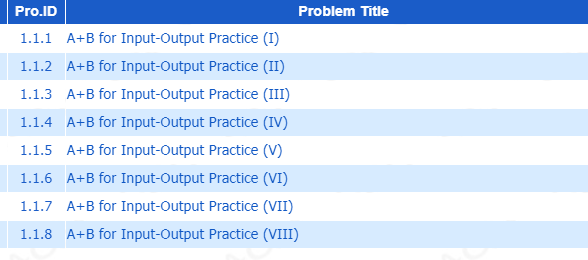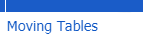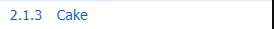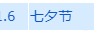# 1-5#include<iostream>
#include<string.h>
using namespace std;
int f;//数组开大些
int main()
{
int T,n,s,t,i;cin>>T;
while(T--)
{
int mmax=0;
memset(f,0,sizeof(f));//标记函数初始化
cin>>n;
while(n--)
{
cin>>s>>t;
if(s>t)swap(s,t);//题目没有明确s，t的关系
//2-3的实际范围是1-4
if(s%2==0)s--;
if(t%2)t++;
for(i=s;i<=t;i++)
f[i]++;
}
for(i=1;i<=400;i++)
mmax=max(mmax,f[i]);
cout<<mmax*10<<endl;
}
}#include<iostream>
using namespace std;
int gcd(int a,int b)//辗转相处法求a，b的最大公约数
{
return a%b==0?b:gcd(b,a%b);
}
int main()
{
int p,q;
while(cin>>p>>q)
{
cout<<p+q-gcd(p,q)<<endl;
}
}#include<iostream>
#include<math.h>
using namespace std;
int main()
{
int t,n,i;cin>>t;
while(t--)
{
int sum=1;
cin>>n;
int m=sqrt(n)+0.5;
for(i=2;i<=m;i++)
if(n%i==0)i==n/i?sum+=i:sum+=i+n/i;//除法可得另一因子
cout<<sum<<endl;
}
}©️2019 CSDN 皮肤主题: 深蓝海洋 设计师: CSDN官方博客